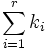Minimum size of generating set is bounded by sum of exponents of prime divisors of order

Statement

Suppose$G$ is a finite group of order$n$, which has prime factorization:$\! n = p_1^{k_1}p_2^{k_2} \dots p_r^{k_r}$

Then, the minimum size of generating set for$G$ is at most equal to:$\sum_{i=1}^r k_i$

Moreover, equality holds if and only if$G$ is a direct product of elementary abelian groups of order$p_i^{k_i}$, i.e.:$G \cong (\mathbb{Z}/p_1\mathbb{Z})^{k_1} \oplus (\mathbb{Z}/p_2\mathbb{Z})^{k_2} \oplus \dots \oplus (\mathbb{Z}/p_r\mathbb{Z})^{k_r}$

Facts used

1. Any generating set of minimum size must be an minimal generating set (in the sense of being irredundant).
2. Size of minimal generating set is bounded by max-length
3. Max-length is bounded by sum of exponents of prime divisors of order

Proof

The proof follows directly by combining Facts (1)-(3).